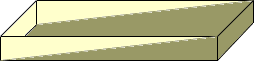Sender: "john e. mcneill" I have an area 20 feet long and 12 feet wide It starts out at a depth of 4 inches and ends up at 0 inches. How much sand do I need to fill the excavation. I beleive this is about 8 or 9th grade level. Hi John, If the hole were 20 feet long by 12 feet wide by 4 inches deep everywhere the volume would be 20x12x(4/12) = 80 cubic feet.But you have a hole of only half this size so its volume is 40 cubic feet. Since you are planning to fill the hole with sand you probrbly want the volume in cubic yards. One cubic yard is 27 cubic feet so you need 40/27 cubic yards which is almost one and a half cubic yards. Cheers, Penny Go to Math Central